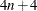### Output Data Sets

PROC CORRESP has two output data sets. The OUTC= data set contains coordinates and the results of the correspondence analysis. The OUTF= data set contains frequencies and other cross-tabulation results.

#### The OUTC= Data Set

The OUTC= data set contains two or three character variables andnumeric variables, where n is the number of axes from DIMENS=n (two by default). The OUTC= data set contains one observation for each row, column, supplementary row, and supplementary column point, and one observation for inertias.

The first variable is named `_TYPE_` and identifies the type of observation. The values of `_TYPE_` are as follows:

• The ‘INERTIA’ observation contains the total inertia in the INERTIA variable, and each dimension’s inertia in the `Contr1``Contrn` variables.

• The ‘OBS’ observations contain the coordinates and statistics for the rows of the table.

• The ‘SUPOBS’ observations contain the coordinates and statistics for the supplementary rows of the table.

• The ‘VAR’ observations contain the coordinates and statistics for the columns of the table.

• The ‘SUPVAR’ observations contain the coordinates and statistics for the supplementary columns of the table.

If you specify the SOURCE option, then the data set also contains a variable `_VAR_` containing the name or label of the input variable from which that row originates. The name of the next variable is either `_NAME_` or (if you specify an ID statement) the name of the ID variable.

For observations with a value of ‘OBS’ or ‘SUPOBS’ for the `_TYPE_` variable, the values of the second variable are constructed as follows:

• When you use a VAR statement without an ID statement, the values are ‘Row1’, ‘Row2’, and so on.

• When you specify a VAR statement with an ID statement, the values are set equal to the values of the ID variable.

• When you specify a TABLES statement, the `_NAME_` variable has values formed from the appropriate row variable values.

For observations with a value of ‘VAR’ or ‘SUPVAR’ for the `_TYPE_` variable, the values of the second variable are equal to the names or labels of the VAR (or SUPPLEMENTARY) variables. When you specify a TABLES statement, the values are formed from the appropriate column variable values.

The third and subsequent variables contain the numerical results of the correspondence analysis.

• `Quality` contains the quality of each point’s representation in the DIMENS=n dimensional display, which is the sum of squared cosines over the first n dimensions.

• `Mass` contains the masses or marginal sums of the relative frequency matrix.

• `Inertia` contains each point’s relative contribution to the total inertia.

• `Dim1``Dimn` contain the point coordinates.

• `Contr1``Contrn` contain the partial contributions to inertia.

• `SqCos1``SqCosn` contain the squared cosines.

• `Best1``Bestn` and `Best` contain the summaries of the partial contributions to inertia.

#### The OUTF= Data Set

The OUTF= data set contains frequencies and percentages. It is similar to a PROC FREQ output data set. The OUTF= data set begins with a variable called `_TYPE_`, which contains the observation type. If the SOURCE option is specified, the data set contains two variables, `_ROWVAR_` and `_COLVAR_`, that contain the names or labels of the row and column input variables from which each cell originates. The next two variables are classification variables that contain the row and column levels. If you use TABLES statement input and each variable list consists of a single variable, the names of the first two variables match the names of the input variables; otherwise, these variables are named `Row` and `Column`. The next two variables are `Count` and `Percent`, which contain frequencies and percentages.

The `_TYPE_` variable can have the following values:

• ‘OBSERVED’ observations contain the contingency table.

• ‘SUPOBS’ observations contain the supplementary rows.

• ‘SUPVAR’ observations contain the supplementary columns.

• ‘EXPECTED’ observations contain the product of the row marginals and the column marginals divided by the grand frequency of the observed frequency table. For ordinary two-way contingency tables, these are the expected frequency matrix under the hypothesis of row and column independence.

• ‘DEVIATION’ observations contain the matrix of deviations between the observed frequency matrix and the product of its row marginals and column marginals divided by its grand frequency. For ordinary two-way contingency tables, these are the observed minus expected frequencies under the hypothesis of row and column independence.

• ‘CELLCHI2’ observations contain contributions to the total chi-square test statistic.

• ‘RP’ observations contain the row profiles.

• ‘SUPRP’ observations contain supplementary row profiles.

• ‘CP’ observations contain the column profiles.

• ‘SUPCP’ observations contain supplementary column profiles.